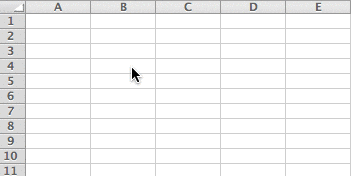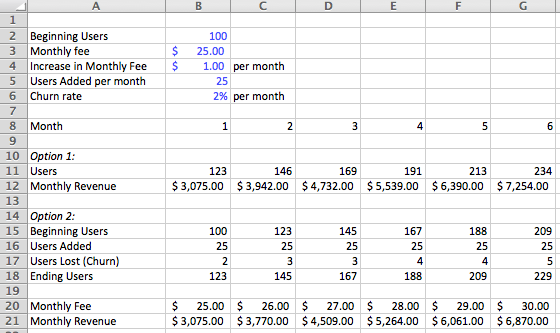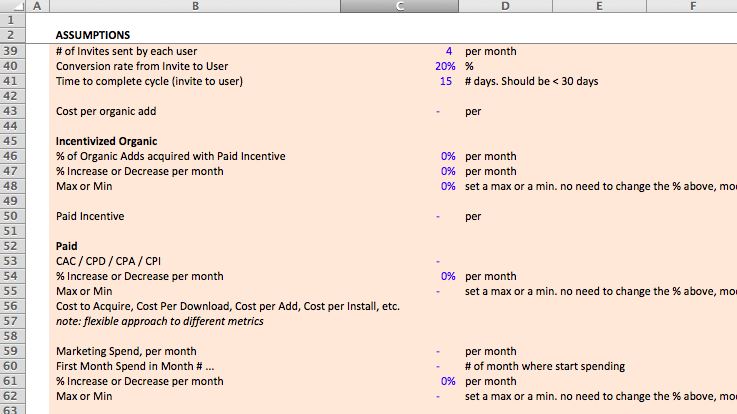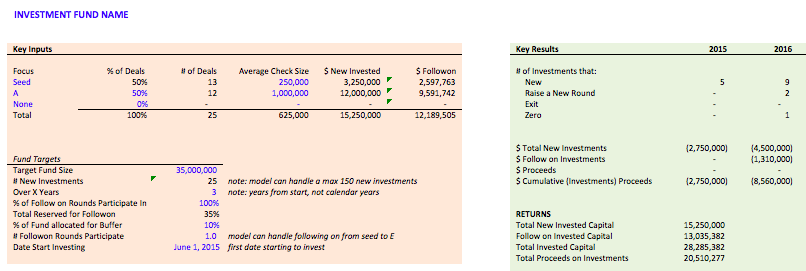# How to Create Assumptions

How to create the assumptions for startup financial models

If you're like 90% of the entrepreneurs I talk to, your first question when you start building your financial model is "what do I input as my assumptions?" Building forward-looking projections for uncertain businesses is by nature difficult: how do we predict how people will adopt or use something that doesn't exist? And without good assumptions, isn't my model useless?

There's a couple ways to address this:

• Research: Use the past performance of other companies as data points for your assumptions. Thankfully, there is more and more data coming to open circles that you can use to benchmark your assumptions: every startup post-mortem has data points, many Quora posts offer data points for comparison, countless blog posts discuss what to expect for a range of rates or metrics. Gather as much data as you can, understand how someone else's experience can inform yours based on difference in product, approach, stage, etc. (and how your experience will likely differ), and use them to benchmark your assumptions.
• Historical metrics: If you have past performance data, that adds potential data points to your forward-looking assumptions. It doesn't provide the exact answers, since future performance may not look like the past, but it's one data point to help you ground your assumptions. And it helps you understand what metrics in your business you'd need to change to make a big breakthrough in performance.
• Make range estimates, not point estimates: Instead of agonizing over whether your conversion rate will be 2% or 5%, focus on the possible range or conversion rates and evaluate the results based upon the range of estimates, not the point estimate of 2% or 5%. Creating a range helps you focus your thinking how the inputs influence the outputs, rather than focusing narrowly on justifying the inputs.

## How do you use assumptions in a model? #

• Use assumptions as variables: Don't hard-code assumptions into formulas. Instead, create your assumptions so that you can easily change an assumption in one place and all formulas and outputs will recalcuate automatically.• Label assumptions clearly: Use descriptive labels so you can understand what assumptions mean. Add notes to your assumptions so you can clearly explain what they mean.
• Organize your assumptions together: There are multiple ways to approach this, but a general best practice is to organize your assumptions together on a single sheet, so that it's easy to see all the assumptions at the same time in a "control center" for your model.
• Show your work. Part of the reason it's best practice to structure assumptions as variables is so that it's easy to change assumptions later with a minimal amount of effort, but another reason is that it helps expose your thinking and structure. This carries itself into the rest of your model: show how your calculations work by breaking your thinking into multiple lines, rather than condense calculations into a single line. In the example below, you can see two approaches to a basic user and revenue buildup: I've laid out a couple assumptions and then calculated users and revenue in two ways. One exposes a lot more information that can lead to better intermediate decision-making, one condenses the calculations and is harder to see the independent impact of changing the assumptions. Note: you'll notice the calculated user and revenue numbers are different, that's because I rounded up the user numbers more granularly for option 2, and that led to a slightly different ending result.In the Standard Financial Model (screenshot below), I organize all of the assumptions for the model on a single sheet, grouping them together by section, label and provide details about each assumption, and use a formatting convention (blue text color) to denote all assumptions. My goal is to easily signify where the assumptions are in the model and what they mean, so that anyone can figure out what's going on as quickly as possible.I also usually create a separate "Key Metrics" section where summarize key inputs and outputs in the model. I'll pull out key metrics that are calculated in other places in the models and report them in the Key Metrics section so that it's easy to see them in one place. I will also create a Key Inputs section next to the Key Metrics to pull out important assumptions from the Assumptions sheet and place them next to the metrics, so that I can easily change key inputs and see how the metrics change, instantly.

In the Venture Fund Model (screenshot below) I have an assumptions page with all the assumptions in the model, but I've pulled out a couple key assumptions - fund size, check size, type of deal, etc. - and placed them next to the performance metrics so I can easily see how the inputs impact the outputs. That helps me understand how key assumptions impact the model and helps me quickly scan to see if a model is performing like I expect it to.Structurally creating assumptions in a model is easy, grounding and justifying assumptions is much harder. Start with a good, clean struture, but don't get hung up on grounding your assumptions perfectly when you start building a model. As you build your model you'll change what assumptions you need, add new assumptions, and find out new data to use. Just focus on continuing to build and understand, and come back to your assumptions once you have the outputs of the model ready to evaluate.

1. You'll notice that I don't necessarily show my work in all places in this model. It's partly because I've tried to make the models a little lighter visually and use less lines for calculations, but it's also because many of the formulas use a mix of index and range functions for a lot of calculations, and it's cleaner for users to see the final result rather than the many intermediate calcs involved in those types of formulas. ↩︎